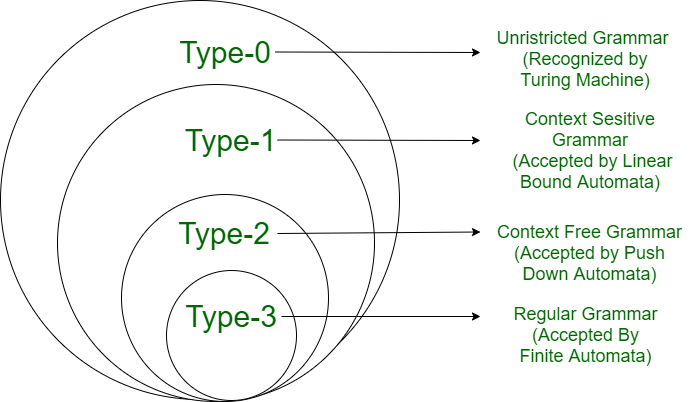Computational Complexity v/s Chomsky Hierarchy

• Last Updated : 20 Oct, 2021

1. Computational complexity :
Computational complexity, a measure of the number of computing resources(time and space) that a particular algorithm consumes when it runs.

The time complexity of a Turing machine is given by the function T(n), where

T(n) = Maximum number of moves made by the TM to process a string of length n.

The space complexity of the Turing machine is given by the function S(n), where

S(n) = Maximum number of tape squares used by the TM for an input of length n.

The time complexity of a simple Turing machine :
Consider the language,

L = {ωcωR  | ω∈(0+1)*}

To recognize a string of the form ωcωR , the TM will require :

Compare first and the last character = 2n + 1 moves

Compare second character from two ends = 2(n -1) + 1 moves

Find the center character c = 1 move

Total number of moves T(n),

= (2n + 1) + (2 (n - 1) +1)+.......(2(n -(n -1)) + 1) + 1
= 2(1+2+.........+n)+n = 2 X (n)(n+1) / 2 + n = n2 + 2n
= n2+2n
= O(n2)

The time complexity can be reduced by taking a two-tape machine.

• The machine copies the input string left of c onto the second tape.
• When c is found on the input tape, the TM moves its second tape head to the left.
• Input tape head continues moving to its right and second tape head to its left.
• The symbols under the two heads are compared as the heads move.
• If all the symbols match and the center character is c then the string is accepted.

The TM makes a maximum of n+1 moves.

T(n) = n+1 = O(n)

The space complexity of TM is given by S(n) = n + 1 [n-string length and one blank symbol]

Non-deterministic time and space complexity :
A non-deterministic TM is of time complexity T(n) if no sequence of choices causes the machine to make more than T(n) moves. Space complexity  is of S(n) if no sequence of choices needs more can S(n) tape cells.

Chomsky Hierarchy :
A grammar can be classified on the basis of production rules. Chomsky classified grammars into following types :Type-3  or Regular Grammar :
A grammar is called type 3 or regular grammar if all its productions are of the following forms:-

A ⇢ ε     A ⇢ a
A ⇢ aB    A ⇢ Ba

where a ∈ ∑ and A, B ∈ V.

A language generated by type-3 grammar is known as regular language.

Type-2 or Context-Free Grammar :
A grammar is called type 2 or context-free grammar if all its productions are of the following form A ⇢ α where  A ∈ V and α ∈ (V ∪ T)*.

V is a set of variables and T is a set of terminals.

The language generated by a type-2 grammar is called a context-free language

Type-1 or Context Sensitive Grammar :
A grammar is called a type-1 or context-sensitive grammar if all its productions are of the following form : α ⇢ β, where β is at least as long as α.

Type-0 or Unristricted Grammar :
Productions can be written without any restriction in unrestricted grammar. If there is a production of the α ⇢ β, then the length of α could be more than the length of β.

• Every grammar is also a number Type 0 grammar.
• Type 2 grammar is also Type 1 grammar.
• Type 3 grammar is also Type 2 grammar.

Computational Complexity v/s Chomsky Hierarchy :

My Personal Notes arrow_drop_up